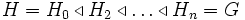# Pronormal and subnormal implies normal

This article gives the statement and possibly, proof, of an implication relation between two subgroup properties. That is, it states that every subgroup satisfying the first subgroup property must also satisfy the second subgroup property
View all subgroup property implications | View all subgroup property non-implications
|
This page describes additional conditions under which a subgroup property implication can be reversed, viz a weaker subgroup property, namely Subnormal subgroup (?), can be made to imply a stronger subgroup property, namely normal subgroup
View other subgroup property implication-reversing conditions
Template:Semi-trivial result

## Statement

### Verbal statement

A subgroup of a group that is both pronormal and subnormal must be normal.

## Facts used

1. Pronormality satisfies intermediate subgroup condition: A pronormal subgroup in the whole group is also pronormal in any intermediate subgroup (this is used in the first proof).

## Proof

The proof involves two steps.

### Pronormal and 2-subnormal implies normal

A 2-subnormal subgroup is a subgroup realized as a normal subgroup of a normal subgroup. We prove that a pronormal 2-subnormal subgroup is normal.

Given:$H \triangleleft K \triangleleft G$, and$H$ is pronormal in$G$.

To prove:$H$ is normal in$G$. Take$g \in G$. We must show that$H^g = H$.

Clearly$H^g$ must lie inside the normal closure of$H$ in$G$, and since$K$ is a normal subgroup containing$H$,$H^g$ must lie inside$K$. Now, since$H$ is pronormal in$G$,$H$ and$H^g$ are conjugate subgroups in$, and thus are conjugate subgroups inside$K$.

This, combined with the fact that$H$ is normal inside$K$, tells us that$H^g = H$.

### Overall proof

The overall proof proceeds by induction, using the above lemma. The induction statement for$n$ says:

Any pronormal subgroup of subnormal depth at most$n$ is normal.

The statement is proved by showing that any pronormal subgroup of subnormal depth$n$ is of subnormal depth at most$n-1$, and then appealing to induction. Here's how. Suppose$H$ is pronormal in$G$ and:$H = H_0 \triangleleft H_1 \triangleleft H_2 \triangleleft \ldots \triangleleft H_n = G$$H$, being pronormal in$G$ is also pronormal in every intermediate subgroup (property-theoretically, pronormality satisfies the intermediate subgroup condition). So$H$ is pronormal in$H_2$. But$H$ is also 2-subnormal in$H_2$, and the previous lemma tells us that any pronormal 2-subnormal subgroup is normal. So$H \triangleleft H_2$, and we get:$H = H_0 \triangleleft H_2 \triangleleft \ldots \triangleleft H_n = G$

Thus$H$ has subnormal depth at most$n-1$, and induction applies.

## Converse

The converse is clearly true: any normal subgroup is pronormal as well as subnormal.